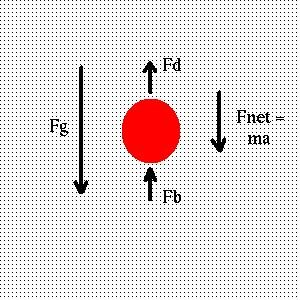# Fluids

The term fluid applies to both liquids and gases. The essential differences between fluids and solids can be summarized as follows:

### Poiseuille's Equation

F = ΔP π r 4 / 8 η l,
where ΔP is the pressure drop experienced by the fluid as a result of viscous losses along the length l of the pipe.

It is necessary to have a single equation which contains exactly two interrelated variables in order to deduce a scaling relationship. In the event that three variables are interrelated through two or more equations, one variable must be eliminated. Any single equation which contains three interrelated variables is insufficient to deduce the relation; in that case a different equation is necessary.

You need a Java-capable browser to be able to use the applets. If they do not work with your Windows system, download the Java VM (Virtual Machine) for your version of Windows at the download section at java.sun.com.

P = ρ g d
This pressure is independent of any atmospheric or surface pressure exerted at depth d = 0.

. . .

Friction often presents itself as drag when an object is moving through a fluid medium. It is a force proportional to the object's velocity, but opposite to the direction of motion:

Fd = - b V.
Here b is the drag coefficient, with dimensions of mass over time. The drag coefficient depends on the radius and shape of the object, and the viscosity of the medium. For a sphere of radius r, the drag coefficient is
b0 = 6 π η r.
For other shapes, we multiply by a shape factor b / b 0 which depends on the shape of the object; for a more cylindrical object, the shape factor might be around 1.5.Opposing gravity is the buoyant force of the water, which attempts to raise the object, and the drag force, which slows its sinking. The buoyant force is equal to the weight of the fluid displaced by the object:

Fb = m v ρ g.
Here v is the specific volume of the object (volume per unit mass). Thus m v gives the volume of the object, and ρ g gives the gravitational force per unit volume of the fluid.
m g - m v ρ g - b V = m A
or
(1 - v ρ) m g - b V = m A
First note that the object floats if v ρ > 1, that is, if the density of the object is less than that of the fluid. If the object does sink, V is initially small, A is positive and V therefore grows. But A clearly decreases, and at some point becomes zero. At that point, V stays constant. The exact result requires calculus, but for large times relative to m / b, the velocity approaches the terminal velocity:
Vt = (1 - v ρ) m g / b.
Note that the hydrostatic pressure does not affect this result, since it exerts a force on the object equally in both the upward and downward directions.

In electrophoresis, which is used in DNA analysis and the determination of protein molecular weights, an electric field takes the place of gravity and buoyancy. The acceleration equation becomes

q E - b V = m A
and the terminal velocity is then
Vt = q E / b
The molecules rapidly reach terminal velocity, and the measurement of the velocity (or correspondingly, the distance the molecules travel in a given time) determines the charge on the molecule. Provided that the molecules have been denatured (unfolded) and charged by the addition of an anionic detergent like sodium dodecylsulfate (SDS), the charge will be proportional to the mass of the molecule and the molecular weight can be determined.

The next section builds an electrical analogy to fluid systems.